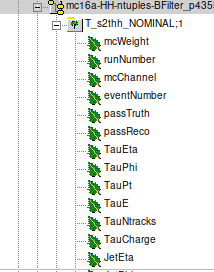# Reading a branch entry in a tree [PyROOT]

Hello Experts,

I am trying to calculate the visible mass of my MC data that is stored in a ntuple, but i am having a problem. I wrote the code below to read the Tau Energy of an event, but it’s returning me zero all the time. The code is the following:

``````histFile = root.TFile.Open(histFileName, "READ")
tree = histFile.Get("T_s2thh_NOMINAL")
f = root.TFile('visiblemass.root', "RECREATE")

TauE = 0
histo = root.TH1F("histo", "My histogram", 100, -5.0, 5.0)
for k in range(tree.GetEntries()):
tree.GetEntry(k)
print(TauE)
histo.Fill( TauE )
histo.Write()
``````

I wrote it based myself in the codes in c++ that i found to read an event. I think the problem may be in TauE = 0, but if i dont declare it, the code wont even run. The same thing if i use TauE = None.

And here is how the tree is formatted:And another question, when i read the TauE entry, and like if i read another EleE (electron energy), could i just sum it with:

TauE+EleE ?

So i would appreciate a help or an reference that can help me. Thank you for your attention.

Hello,

Can you try with:

``````histFile = root.TFile.Open(histFileName, "READ")
tree = histFile.Get("T_s2thh_NOMINAL")
f = root.TFile('visiblemass.root', "RECREATE")

histo = root.TH1F("histo", "My histogram", 100, -5.0, 5.0)
for entry in tree:
print(entry.TauE)
histo.Fill( entry.TauE )
histo.Write()
``````

Please note that you are iterating over the tree in Python, which can be slow for big datasets (we only recommend it for small exploratory work). If you have to do bigger computations, RDataFrame runs the event loop in C++ (which is much faster) and can exploit all the cores of your machine.

Regarding the sum of the two branches (`TauE+EleE`), you would need to do:

``````res = entry.TauE + entry.EleE
``````

inside the loop.

1 Like

Hi, i am getting an error when trying to run this. This is the error:

File “visiblemass.py”, line 73, in
histo.Fill( entry.TauE)
TypeError: none of the 3 overloaded methods succeeded. Full details:
int TH1::Fill(double x) =>
TypeError: could not convert argument 1 (must be real number, not vector)
int TH1::Fill(const char* name, double w) =>
TypeError: takes at least 2 arguments (1 given)
int TH1::Fill(double x, double w) =>
TypeError: takes at least 2 arguments (1 given)

And how would this work in RDataFrame? Something like this?

``````df = root.RDataFrame("T_s2thh_NOMINAL", histFileName)
df = df.Define("z", "sqrt(TauE*TauE - TauPt*TauPt)")
df.Display().Print()
df.Snapshot('tree', 'df032_MakeNumpyDataFrame.root')
``````

But i don’t really know how .Define() works, does it run over all events? And i want to make some cuts in the energy and pt, and i think the number of entries in both branches will be different , so .Define() wont work, right?

Hi,

Hi, i am getting an error when trying to run this. This is the error:

What is the type of `entry.TauE` ? Can you do `print(type(entry.TauE))`?

And how would this work in RDataFrame? Something like this?

Yes that looks good, congrats!

But i don’t really know how .Define() works, does it run over all events?

`Define` will tell RDataFrame to run the expression you pass `sqrt(TauE*TauE - TauPt*TauPt)` for every event in the tree and define a new column with the result.

And i want to make some cuts in the energy and pt, and i think the number of entries in both branches will be different , so .Define() wont work, right?

You can do cuts with `Filter`. So you can `Filter` first and then `Define`, and `Define` will only be applied to the events that passed the filter.

1 Like

HI, so i did the print(type(entry.TauE)) and the output was:

<class cppyy.gbl.std.vector at 0x8e8d390>

Hello,

It seems that `entry.TauE` is not a double but a vector, now it depends on what you want to do. You want to fill the histogram with all the elements of that vector? If so, you have to iterate on `entry.TauE`:

``````for elem in entry.TauE:
histo.Fill(elem)
``````
1 Like

Oh, that makes sense, thank you! But, when i use .Define() and:

``````df = df.Define("z", "sqrt(TauE*TauE - TauPt*TauPt)")
``````

Does it work properly, being that TauE and TauPt are vectors?

Yes that should work because RDataFrame reads std::vector branches as `RVec`s, which is a ROOT class:

https://root.cern/doc/master/classROOT_1_1VecOps_1_1RVec.html

that allows these vector operations.

1 Like

Indeed, i calculated the sqrt( TauETauE-TauPtTauPt) both ways and the result were similar. But, when i loop trough the events without RDataFrame and fill the histogram, i get less entries compare to the histogram using the Histo1D method. shouldn’t it be equal?

Here’s my code:

``````histo = root.TH1F("histo", "My histogram", 100, 0.0, 300000.0)
for entry in tree:
if(entry.TauPt and entry.TauE):
taue = 0
for elem in entry.TauE:
taue = taue + elem
taupt = 0
for elem in entry.TauPt:
taupt = taupt + elem
histo.Fill(sqrt(taue*taue-taupt*taupt))
histo.Write()
``````

Using the .Histo1D() the histogram has 383071 entries, and using the code above, it has only 300344.

What was the filter you used in RDataFrame? In the explicit Python loop version above, it seems you are just checking that both vectors have at least one element.